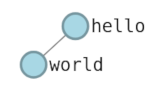# Hello World

The hello world topology is a network of two interconnected nodes named `hello` and `world`.import mergexp as mx
# create a topology named 'hello-world'
net = mx.Topology('hello-world')
# define two nodes hello and world
hello = net.device('hello')
world = net.device('world')
# connect hello and world
# access endpoint by link operator
# specify the net object as the experiment topology created by this script
mx.experiment(net)

This example shows the 3 basic modeling components in the Merge experiment modeling library

• Topology: contains devices interconnected by links.
• Device: a member of a network.

## Topologies#

A `topology` object is the structural model of an experiment. It contains the devices in an experiment and the `links` that interconnect them. `Topology` constructors take a single argument that is the name of the topology.

net = mx.Topology('hello-world')

## Devices#

`Device` objects are the nodes in your experiment network. In this case we have two `devices` 'hello' and 'world'. This example shows the simplest way to create a device, which is just to give it a name. Later examples will show how constraints can be used to specify specific property requirements about devices such as number of CPU cores and amount of memory.

hello = net.device('hello')
world = net.device('world')

`Link` objects interconnect devices. The `link` constructor takes in a list of devices as the only required parameter. In this example we only provide two devices, so what we wind up with is a point to point `link`. Two is the minimum number of devices that may be supplied to a `link`, however there is no upper limit on the number of devices. `Links` with more than two devices are called multipoint `links` and are explained in a later example.

Like devices links can also take constraint based properties such as delay and capacity limiting. Later examples will demonstrate link modeling in detail.

One thing to notice about this topology is how IP addresses are assigned to nodes. IP addresses are assigned to `endpoint` objects, and the number of `endpoints` a given node has depends on how many `links` are connected to it. In this example we created a single `link` between the two nodes, so each node has one `endpoint`. We can access the `endpoint` through the `link` object by indexing over the node, this is demonstrated in the example code as

Notice also that IP addresses are assigned to `endpoints` as lists, so there can be any number of IP addresses assigned to a given `endpoint`.